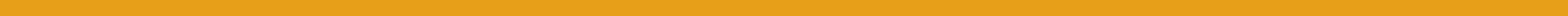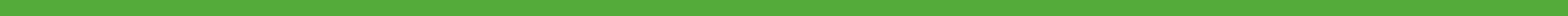# What’s new? May 2020

2019-20: Sep | Oct | Nov | Dec | Jan | Feb | Mar | Apr#### Videos

We have continued to add videos for the GCSE topics. As mentioned last month, our videos do not contain any adverts and are recorded in 16:9, which makes far better use of screen space than 4:3 videos that still seem to get a lot of views. Some modern phones have even more elongated aspect ratios than 16:9, and 4:3 videos on these devices only use the middle third of the screen! Our videos are also all recorded in either 1080p or 4k.#### KS3-4 applets

We are continuing to work on guidance fading applets to give students the chance to practise randomly generated questions and reveal step-by-step solutions. For example, this applet at A9c is about finding the equation of a line through a given point with a given gradient or the equation of a line through two given points.

##### Rounding: principle vs procedure

When it comes to rounding, students are often taught a procedure involving checking if the next digit is is < or ≥ 5. An over-emphasis on this procedure can leave students easily thrown if they are asked to round a number to the nearest n, where n is not a power of 10. For example, imagine if students are asked to round 17 to the nearest 5 or nearest 6. This applet is designed to focus on the principle of rounding.

##### Standard form

We have added “skills isolation” question generators at N9a. N9b, and N9c. For example, this applet allows students to practise converting a number in standard form into a number involving a different power of 10, which is a useful thing to be able to do, especially when it comes to adding or subtracting numbers in standard form.

##### Quartiles

It is often easier to give students an accurate understanding of quartiles using a diagram than using words alone. For example, consider which, if any, of the following statements are true. And even if any are true, how well does the statement alone convey to a student what a lower quartile is?

• Exactly 25% of the values in a data set are less than the lower quartile
• At least 25% of the values in a data set are less than the lower quartile
• At most 25% of the values in a data set are less than the lower quartile
• Exactly 25% of the values in a data set are less than or equal to the lower quartile
• At least 25% of the values in a data set are less than or equal to the lower quartile
• At most 25% of the values in a data set are less than or equal to the lower quartile

This applet at S4e is designed to show how quartiles split—but not necessarily partition—a set into four equally-sized subsets. Students will have previously encountered that the idea of the median is to partition a data set into two halves, but of course this isn’t strictly possible if the data set contains an odd number of values. Nevertheless, whether the data set has an odd or even number of values, if listed in order, there will be an equal number of values to the left of the median and to the right. Note that some values to the left and/or right might be equal to the median; but the number of values to the left and right will be equal. You can see this in the applet below. Drag the slider to change the number of values in the data set.

The idea of quartiles is to partition a data set into four quarters. Again, this isn’t strictly possible unless the number of values in the set is a multiple of 4. We can however do a pretty good job as follows:

1. Make sure the values are in order, and find the median.
2. Look at the values to the left of the median, and find the median of these. This gives you the lower quartile.
3. Look at the values to the right of the original median, and find the median of these. This gives you the upper quartile.

By doing this, the following will all be equal:

• the number of values to the left of the lower quartile,
• the number of values between the lower quartile and the median,
• the number of values between the median and the upper quartile, and
• the number of values to the right of the upper quartile.